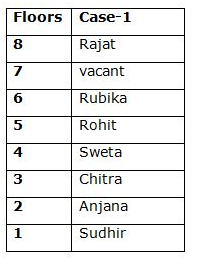# SBI Clerk Prelims Reasoning Ability Questions 2021 (Day-53)

Dear Aspirants, Our IBPS Guide team is providing new series of Reasoning Questions for SBI Clerk Prelims 2021 so the aspirants can practice it on a daily basis. These questions are framed by our skilled experts after understanding your needs thoroughly. Aspirants can practice these new series questions daily to familiarize with the exact exam pattern and make your preparation effective.

Start Quiz

Order and ranking

Direction (1-3): Study the following information carefully and answer the questions given below it.

There are 36 students in the class. In exam each student got different rank. G got 24th rank from bottom. L got 23rd rank from top. S got 31st rank from top. Rank of K is exactly between G’s rank and L’s rank.

1)
What is the rank of K from top?

A.19th

B.18th

C.20th

D.21st

E.None of these

2) What is the rank of L from bottom?

A.16th

B.17th

C.15th

D.14th

E.None of these

3) How many student ranks are between rank of K and rank of S?

A.12

B.11

C.13

D.10

E.None of these

Inequality

Direction (4-5):  In each of the following questions, relationship between different elements is shown in the statements followed by two conclusions. Find the conclusion which is definitely true.

a)If only conclusion I follows.

b)If only conclusion II follows.

c)If either conclusion I or II follows.

d)If neither conclusion I nor II follows.

e)If both conclusions I and II follow.

4) Statements:

S ≥ N > P < Q = B ≤ X; Z ≥ L ≤ S

Conclusion:

I)L < X

II)P ≤ B

5) Statements:

M < B ≥ H > S; P ≥ F ≤ A < H; F ≤ C = V

Conclusion:

I)C ≥ B

II)V < B

Floor based puzzle

Direction (6-10):  Study the following information carefully and answer the questions given below it.

There are seven persons namely Anjana, Chitra, Rajat, Rohit, Rubika, Sudhir and Sweta are living in an eight story building and there is a vacant floor. In the building ground floor is numbered as floor number one, above it floor number two and so on. Similarly top floor is numbered as floor number eight.

Rubika lives just below of the vacant floor. Vacant floor is on odd number floor. There are three floors gap between Rubika and Anjana. Sweta does not live on top floor. There is one floor gap between Sweta and Anjana. There are two floors gap between Rajat and Rohit. Rajat lives above of Rohit. Sudhir lives on odd number floor below of Anjana. There are at least two floors gap between Rajat and Chitra.

6) Chitra lives on which floor number?

A.Two

B.One

C.Three

D.Seven

E.None of these

7) How many persons are living above of Rohit?

A.One

B.Two

C.Three

D.Six

E.None of these

8) Who among the following lives just above of the vacant floor?

A.Sweta

B.Chitra

C.Rohit

D.Rajat

E.None of these

9) Who lives on floor number six?

A.Rubika

B.Sweta

C.Anjana

D.Rohit

E.None of these

10) How many floors gap between Rajat and Sweta?

A.One

B.Two

C.Three

D.Four

E.None of these

Directions (1-3):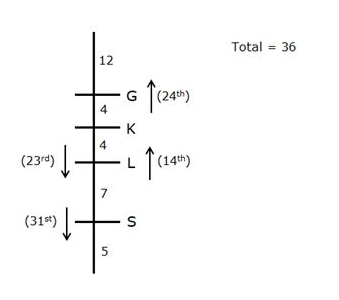Directions (6-10) :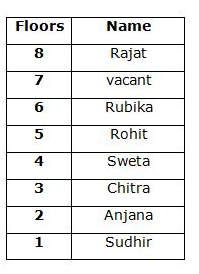Rubika lives just below of the vacant floor. Vacant floor is on odd number floor.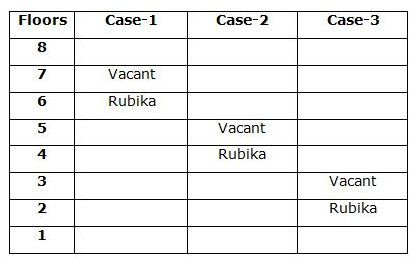There are three floors gap between Rubika and Anjana.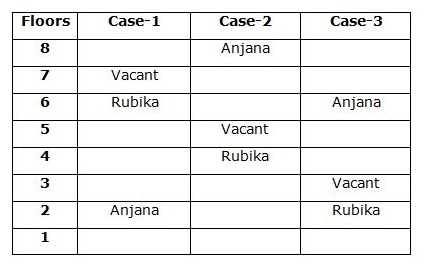Sweta does not live on top floor. There is one floor gap between Sweta and Anjana.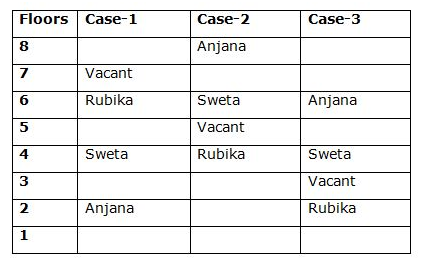There are two floors gap between Rajat and Rohit. Rajat lives above of Rohit. Here, Case-2 is eliminated.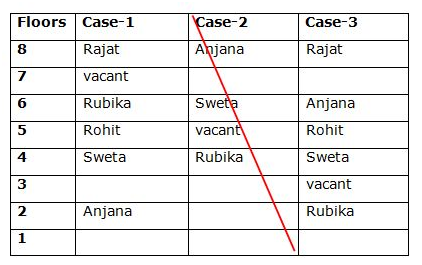Sudhir lives on odd number floor below of Anjana.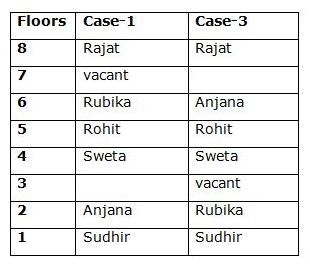There are at least two floors gap between Rajat and Chitra. Here, Case-3 is eliminated.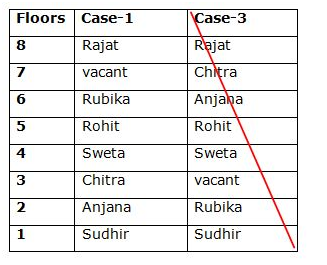Hence, Final solution is Case-1.